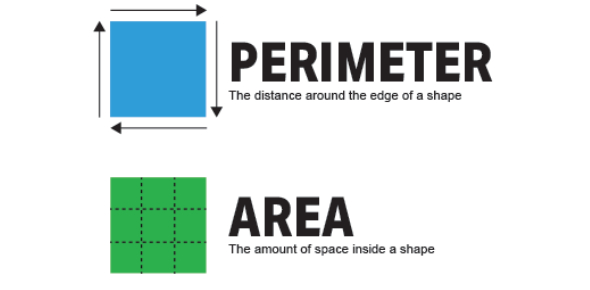# Perimeter And Area

10 Questions | Total Attempts: 5250SettingsMath Unit 8

• 1.
Understanding area and perimeter can help me to know what it will cost me to put a new floor in my home or put a fence around my yard.
• A.

True

• B.

False

• 2.
Why might you want to measure the area?
• A.

To know how much it will cost me to put carpet in my home

• B.

To know how much grass seed I need for my yard

• C.

All of the above

• D.

None of the above

• 3.
What is the area of the figure?
• A.

4

• B.

8

• C.

2

• 4.
Which figure has an area of 5? a.        b.      c.
• A.

A

• B.

B

• C.

C

• 5.
Which number sentence represents the area of the figure?
• A.

3 + 6 =

• B.

3 x 6 =

• C.

3 + 6 + 3 + 6 =

• 6.
Which number sentence represents the area of the figure?
• A.

2 + 4 =

• B.

4 + 4 =

• C.

4 x 2 =

• 7.
What is the perimeter of the figure?
• A.

12

• B.

4

• C.

4 x 2

• 8.
Which figure has a perimeter of 16?a.      b.      c.
• A.

A

• B.

B

• C.

C

• 9.
Which number sentence represents the perimeter of the figure?
• A.

4+6+4+6 =

• B.

4 + 6 =

• C.

6 x 4 =

• 10.
For which figure would the perimeter be solved by the number sentence 4+6+4+6?a.    b.     c.
• A.

Figure a

• B.

Figure b

• C.

Figure c

Related TopicsBack to top